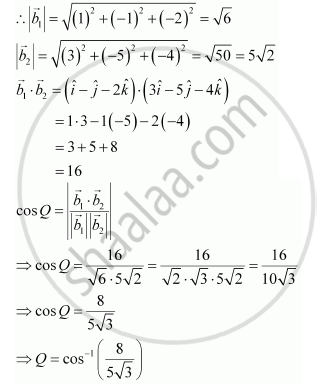Share

# Find the Angle Between the Following Pairs of Lines: Vecr = 3hati + Hatj - 2hatk + Lambda(Hati - Hatj - 2hatk) and Vecr = 2hati - Hatj -56hatk + Mu(3hati - 5hatj - 4hatk) - CBSE (Science) Class 12 - Mathematics

#### Question

Find the angle between the following pairs of lines:

vecr = 3hati + hatj - 2hatk + lambda(hati - hatj - 2hatk) and vecr = 2hati - hatj -56hatk + mu(3hati - 5hatj - 4hatk)

#### Solution

The given lines are parallel to the vectors, vecb_1 = hati - hatj - 2hatk and vecb_2 = 3hati - 5hatj - 4hatk, respectively.Is there an error in this question or solution?

#### APPEARS IN

NCERT Solution for Mathematics Textbook for Class 12 (2018 to Current)
Chapter 11: Three Dimensional Geometry
Q: 10.2 | Page no. 478

#### Video TutorialsVIEW ALL 

Solution Find the Angle Between the Following Pairs of Lines: Vecr = 3hati + Hatj - 2hatk + Lambda(Hati - Hatj - 2hatk) and Vecr = 2hati - Hatj -56hatk + Mu(3hati - 5hatj - 4hatk) Concept: Angle Between Two Lines.
S